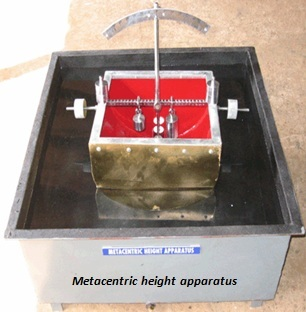Feb 26, 2016

Feb 26, 2016

Feb 26, 2016

# To Determine The Metacentric Height Of a Ship Model

## Apparatus:

1. Water bulb
2. Metacentric height apparatus
3. Scale or measuring tube

## Concepts:

### Metacenter:

When a floating body is given a small displacement it will rotate about a point, so the point at which the body rotates is called as the Metacenter.
“OR”
The intersection of the lines passing through the original center of buoyancy and center of gravity of the body and the vertical line through the new center of buoyancy.

### Metacentric height:

The distance between center of gravity of a floating body and Metacenter is called as Metacentric height.

### Why to find Metacentric height?

It is necessary for the stability of a floating body, Ifmetacenter is above center of gravity body will be stable because the restoring couple produced will shift the body to its original position.

### Center of buoyancy:

The point though which the force of buoyancy is supposed to pass is called as the center of buoyancy.
“OR”
The center of area of the immersed portion of a body is called its center of buoyancy.

## Procedure:

1. First of all I adjust the movable weight along the vertical rod at a certain position and measured the distance of center of gravity by measuring tape.

2. Then I brought the body in the water tube and changed the horizontal moving load distance first towards right.

3. The piston tilted and suspended rod gave the angle of head, I noted the angle for respective displacements.

4. I did the same procedure for movable mass by changing its position towards left.

5. Then I took the body from water tube and find another center of gravity by changing the position of vertically moving load.

6. I again brought the body in the water tube and find the angle of head by first keeping the movable load towards right and then towards left.

7. I repeated the above procedure for another center of gravity.

8. I calculated the metacentric height by the following formula:

MH = w * d / W * tanØ

Where
MH = Metacentric height
w = Horizontally movable mass
d = Distance of movable mass at right or left of center
W = Mass of assemble position
Ø = Respective angle of heel

## Observationcs & Calculations:

Horizontally movable mass = w = 0.31kg
Mass of assemble position = W = 1.478kg
Center of gravity = y1 = 8 mm
Center of gravity = y2 = 9 mm
Center of gravity = y3 = 10 mm

Considering Right Portion

 S.No Distance of movable mass at right of center (mm) Y1 Angle of head "Ѳ" Y2 Y3 Y1 Metacentric height(MH) Y2 Y3 01 20 2.5 2.75 3.3 96.07 87.83 72.75 02 40 4.5 5.5 6 106.6 87.13 79.82 03 60 7.5 9 9.5 95.58 79.45 75.20

Considering Left Portion

 S.No Distance of movable mass at left of center (mm) Y1 Angle of head”Ѳ” Y2 Y3 Y1 Metacentric height (MH) Y2 Y3 01 20 2.5 2.75 3.3 96.07 87.83 72.75 02 40 4.5 5.5 6 106.6 87.13 79.82 03 60 7.5 9 9.5 95.58 79.45 75.20